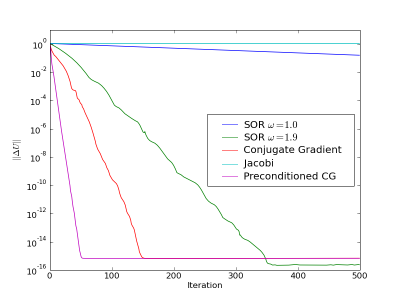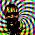## Sunday, March 1, 2009

### Three Dimensional Poisson's Equation

This is a follow up to the post I wrote a while back about implementing numerical methods. The goal is to demonstrate a work-flow using tools that come included in the Fedora Linux (and many other) distributions.
1. In Maxima
• Define governing equations
• Substitute finite differenceexpressions for differential expressions in the governing equations
• Output appropriate difference expressions to Fortranusing f90()
2. In Fortran: wrap the expression generated by Maxima in appropriate functions, subroutines and modules

3. In Python
• Compile modules with f2py, which comes packaged along with numpy
• Write the high-level application code, parsing input decks, performing optimization, grid convergence studies, or visualizations that use the compiled Fortran modules

### Maxima

The governing equation is the three-dimensional Poisson's equation.In Cartesian coordinatesThe Maxima code to define this equation is straightforward:
depends(u,x); depends(u,y); depends(u,z); /* Poisson's equation: */ p : 'diff(u,x,2) + 'diff(u,y,2) + 'diff(u,z,2) = -f;
Now that we have the continuous differential equation defined we can decide on what sort of discrete approximation we are going to solve. The simple thing to do with second derivatives is to use central differences of second order accuracy, which require only information from closest neighbours in the finite difference grid. Replacing the differential expressions with difference expressions is accomplished in Maxima with the ratsubst() function.
/* substitue difference expressions for differential ones: */
p : ratsubst((u[i-1,j,k] - 2*u[i,j,k] + u[i+1,j,k])/dx**2 , 'diff(u,x,2), p);
p : ratsubst((u[i,j-1,k] - 2*u[i,j,k] + u[i,j+1,k])/dy**2 , 'diff(u,y,2), p);
p : ratsubst((u[i,j,k-1] - 2*u[i,j,k] + u[i,j,k+1])/dz**2 , 'diff(u,z,2), p);
/* substitute the correct array value of f:*/
p : ratsubst(f[i,j,k], f, p);
This assumes that the solution u and the forcing function f are stored in three dimensional arrays, if not then the indexing in the difference expressions becomes more complicated. If we want to apply a Gauss-Seidel method to solve our elliptic problem we just need to solve p for u[i,j,k], and then dump that expression to Fortran format.
gs : solve(p, u[i,j,k]);
del : part(gs,2) - u[i,j,k];
/* output for fortran: */
with_stdout("gs.f90", f90(d = expand(del)));
This gives the expression for the update to the solution at each iteration of the solver.

### Fortran

The Maxima expressions developed above need control flow added so they get applied properly to our solution array.
do k = 1, nz
do j = 1, ny
do i = 1, nx
d = (dy**2*dz**2*u(i+1,j,k)+dx**2*dz**2*u(i,j+1,k)+dx**2*dy**2* & u(i,j,k+1)+dx**2*dy**2*dz**2*f(i,j,k)+dx**2*dy**2* & u(i,j,k-1)+dx**2*dz**2*u(i,j-1,k)+dy**2*dz**2*u(i-1,j,k))/ & ((2*dy**2+2*dx**2)*dz**2+2*dx**2*dy**2)-u(i,j,k) u(i,j,k) = u(i,j,k) + w*d
end do
end do
end do
The expression for the update was generated from our governing equations in Maxima, one of the things to notice is the  w  that multiplies our update, this is so we can do successive over relaxation. Also, we'll go ahead and package several of these iterative schemes into a Fortran module
module iter_schemes
implicit none
contains

...bunches of related subroutines and functions ...

end module iter_schemes

### Python

Now we want to be able to call our Fortran solution schemes from Python. Using F2py makes this quite simple:
[command-line]\$ f2py -m iter_schemes -c iter_schemes.f90
This should result in a python module iter_schemes.so that can be imported just as any other module.
import numpy
from iter_schemes import *

... do some cool stuff with our new module in Python ...

### Conclusion

This approach might seem like over-kill for the fairly simple scalar equation we used, but think about the complicated update expressions that can be generated for large vector partial differential equations like the Navier-Stokesor Maxwell's equations. Having these expressions automatically generated and ready to be wrapped in loops can save lots of initial development and subsequent debugging time. It also makes generating unit tests easier, and so encourages good development practice. But does it work? Of course, here's the convergence of the Gauss-Seidel and SOR schemes discussed above along with a Jacobi scheme and a conjugate gradient scheme applied to a 50 by 50 by 50 grid with a manufactured solution.The plot was generated with the Python module matplotlib.

1.2.Dear jstults,

Hi,

Hope all is fine with you.

In the first I'm very thankfull for your really valuable materials about solving Poisson's equation in three dimensions, numeerically.

Would you mind Mail me via Phymalidoust@gmail.com for more discussions, please?

Bests,

3.Just post your question; that way other folks can benefit from the discussion. There's probably plenty of folks who have the same or similar questions.

2-D simplifies things a bit so they are good example problems to talk about.

Fire away...

4.What sort of discretization did you use? What does the discrete system matrix (or expression) that you came up with look like?

The Thomas algorithm (Gaussian elimination for a tridiagonal system) only works for a 1-D problem. You'll have to use relaxation or some other iterative method; or if the problem is small enough just get Matlab to solve the system for you (this is easiest when you are learning).

Once you have inverted the small problem directly with Matlab, then you can check that your iterative code is giving (roughly) the same answer.

The other tip I would give you is to break up your solution into components that can be tested individually.

Write a routine that calculates your forcing function, and test that it is operating correctly.

Write a routine that calculates the derivatives, and test that it is operating correctly.

Write a routine that takes the forcing function and derivatives and performs your chosen iterative update, and test that it is operating correctly. For example, you can check to see that it converges to the "direct" solution that Matlab gave you.

If you follow this sort of methodical approach you should be able to locate which part of your solution method (derivative calculation, forcing function, iterative update) is incorrect.

5.I don't have time to debug your code. If you break the solution up into chunks and unit test those chunks, then you probably won't need me to look at your code because you will have located the problem yourself.

I'd be happy to help once you identify a specific "part" that is broken, and you aren't sure how to fix it. Finding the broken part is a useful experience for you, and I wouldn't want to rob you of it.

6.It is simply a collaboration if you are willing.

We can help and write a paper (ISI), including Numerical parts and also analytical.
In effect it seems that solving of this PDE and relevanted initial conditions is too hard for me. I tried but couldn't....

Please let me know, what do you think about collaboration in this project?

7.Thank you, I appreciate your offer, but I don't think this lines up with my current research interests.

I think it is good to get these posts up though, perhaps one of the other readers will be interested (this is the most read post on my site, ~150 views/month), and they can contact you through your profile.

8.O.K. Grate.

Really thanks for your interesting discussion. I just wait for a professional person in this Field.
9.10.